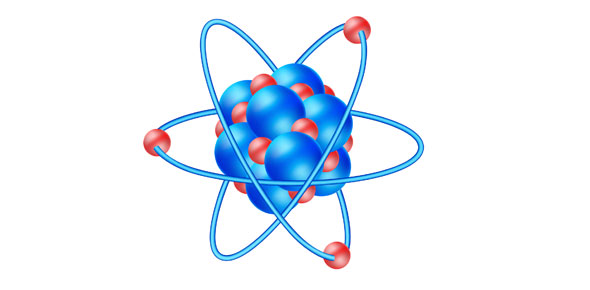# Quiz Over Atomic Structure Of An Atom

10 Questions | Total Attempts: 53SettingsA quiz to assess your understanding.

• 1.
An atom has 6 protons. How many electrons does it have?
• A.

8

• B.

4

• C.

6

• D.

0

• 2.
What particles are found in a nucleus of an atom?
• A.

Protons only

• B.

Neutrons only

• C.

Electrons, protons and neutrons

• D.

Protons and neutrons

• 3.
An ion M2+ contains 12 protons. The electronic configuration in the atom M is
• A.

[Ne]

• B.

[Ne]3s2

• C.

[He]3s2

• D.

[Ne]3p2

• 4.
Which of these statements correctly describes an electron shell with the principal quantum shell n=2?1  A total of 8 electrons can be accomodated in this shell.2  An orbital in this shell may be either sperical or dumb-bell in shape.3  Electrons occupy its orbitals starting with that of lower energy first.
• A.

1,2 and 3 are correct.

• B.

1 and 2 only are correct

• C.

2 and 3 only are correct

• D.

1 only is correct

• 5.
What is the proton number of an element that has 3 unpaired electrons in its ground state?
• A.

14

• B.

16

• C.

15

• D.

26

• 6.
What is the shape of a p orbital?
• A.

Peanut

• B.

Figure of 8

• C.

Hour glass

• D.

Dumb-bell

• 7.
The first seven ionization energies of an element are as follows (in kJ/mol):790, 1600, 3200, 4400, 16100, 19800, 23800What is the likely proton number of this element?
• A.

1

• B.

6

• C.

8

• D.

9

• 8.
Which of the following particle is isoelectronic with the ion formed by a magnesium atom?
• A.

Oxygen atom

• B.

LiF

• C.

Si

• D.

Oxide ion

• 9.
Which property is the same for the two atoms 40Ar and 40K?
• A.

The number of electrons

• B.

The number of neutrons

• C.

The number of nucleons

• D.

The number of protons

• 10.
Which of the following elements has the highest first ionization energy?
• A.

Carbon

• B.

Oxygen

• C.

Sodium

• D.

Sulfur

Related TopicsBack to top